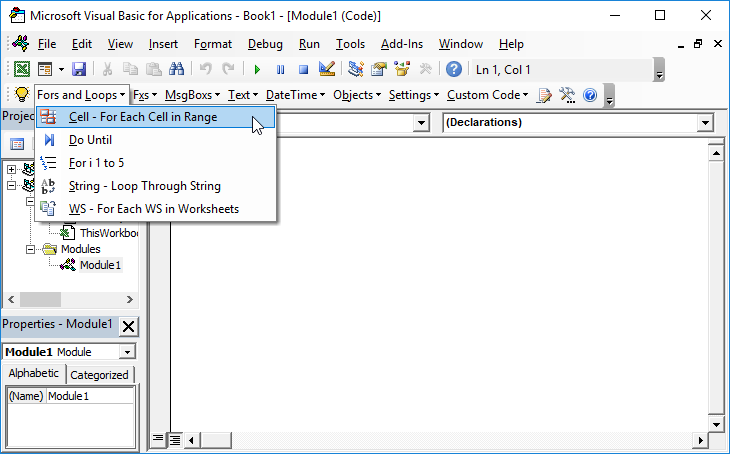# VBA For Loop – Loop Through a Range of Cells

In VBA, you can loop through a range of cells, applying actions to each cell in the range.

If you want to test a condition for each cell in a range using VBA, the best way is to loop through the range, testing each cell. Here are two code examples to demonstrate how to loop through a range. You can replace the range value for whatever range you need to loop through.

## Loop Through Range of Cells

This example will loop through a range of cells. The if statement tests the condition if the cell contains the text “FindMe” and shows a message box with the location of the text if found.

``````Public Sub LoopCells()

Dim c As Range

For Each c In Range("A1:A10")
If c.Value = "FindMe" Then
MsgBox "FindMe found at " & c.Address
End If
Next c

End Sub
``````

## Loop Through Entire Column

This example loops through the entire column A range.

``````Public Sub LoopColumn()

Dim c As Range

For Each c In Range("A:A")
If c.Value = "FindMe" Then
MsgBox "FindMe found at " & c.Address
End If
Next c

End Sub
``````

## Loop Through Entire Row

The next example loops through row 1 considering the entire row a range.

``````Public Sub LoopRow()

Dim c As Range

For Each c In Range("1:1")
If c.Value = "FindMe" Then
MsgBox "FindMe found at " & c.Address
End If
Next c

End Sub``````

Stop searching for VBA code online. Learn more about AutoMacro – A VBA Code Builder that allows beginners to code procedures from scratch with minimal coding knowledge and with many time-saving features for all users!